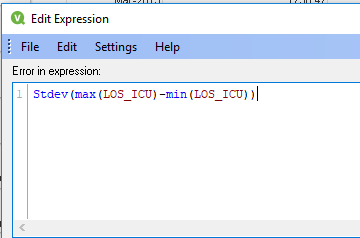# New to QlikView

Discussion board where members can get started with QlikView.

New Contributor

## standard deviation calculation

I have a table, see below. For each month, the minimum value is subtracted from the maximum value for that month to get a range (see formula in table).  The range for Jan-2015 is 2,031.  I want to calculate the standard deviation of the entire second column, the one that has the range of each month.  In the expression tab under "total mode" I can show the standard deviation at the top of the column, like it is now.  The std dev is 2,448.  But I want to be able to create a variable or field that calculates this for me.  Please help!1 Solution

Accepted Solutions
Valued Contributor II

## Re: standard deviation calculation

you are right,

it's not possible to have nested stdev and max/min

I tried with aggr, that works :

Stdev( aggr(max(los_icu),YearMonth)) - aggr(min(los_icu),YearMonth))

7 Replies
Valued Contributor II

## Re: standard deviation calculation

Hi,

stdev(max(los_ico)-min(los_ico)) doesn't work ?

Daniel where is the problem exactly ?

regards

Highlighted
New Contributor

## Re: standard deviation calculation

Hi Oliver, see the screen shot below.  It is telling me there is an error in my expression.Valued Contributor II

## Re: standard deviation calculation

you are right,

it's not possible to have nested stdev and max/min

I tried with aggr, that works :

Stdev( aggr(max(los_icu),YearMonth)) - aggr(min(los_icu),YearMonth))

New Contributor

## Re: standard deviation calculation

Nothing is populating, I typed in that formula exactly....see below.Valued Contributor II

## Re: standard deviation calculation

in the new column you have 2448 as total : it's not that ?

New Contributor

## Re: standard deviation calculation

i got it, thanks!

Valued Contributor II

## Re: standard deviation calculation

thanks

if you want it same column above,

use function : dimensionality()

= 0 it's the header subtotal

= 1 it's a row